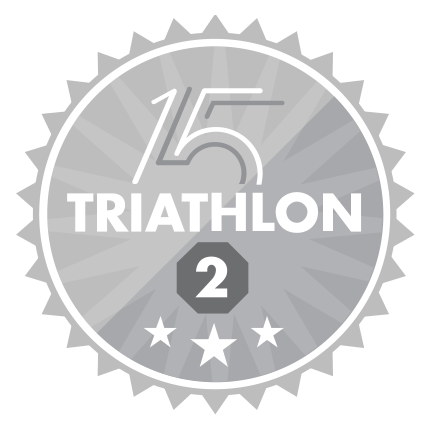Community Profile# Jang geun Choi

### Pusan national university

Last seen: 26 days ago Active since 2014

I'm a student studying ocean science using matlab.

#### Statistics

All
••••••••••••#### Content Feed

View by

Submitted

Physical oceanography: wave and energy conservation
Calculate wave and its energySubmitted

Physical oceanography: wave equation (two-dimensional)
Simulate two-dimensional waveSubmitted

Physical oceanography: wave equation (open boundary)
Simulate wave in the open boundarySubmitted

Physical oceanography: wave equation
Simulate simple waveSubmitted

Physical oceanography: geostrophic current (numerical)
Calculate geostrophic current using numerical methodSubmitted

Physical oceanography: geostrophic current (analytical)
Show theory of geostrophic currentSubmitted

Physical oceanography: Inertial oscillation (semi-implicit)
Simulate Inertial oscillation using semi-implicit methodSubmitted

Physical oceanography: Inertial oscillation (implicit)
Simulate Inertial oscillation using implicit methodSubmitted

Physical oceanography: Inertial oscillation (explicit)
Simulate Inertial oscillation using explicit methodSubmitted

Physical oceanography: diffusion
Simulate diffusionSubmittedSubmitted

Physical oceanography: numerical analysis 2
Solve equation using numeric methodSubmitted

Physical oceanography: numerical analysis 1
Solve equation using numeric method